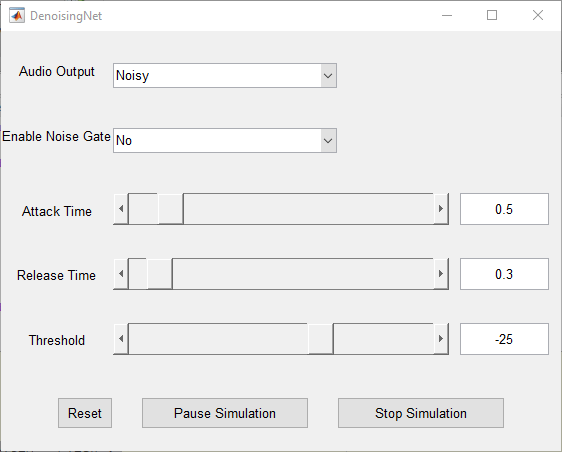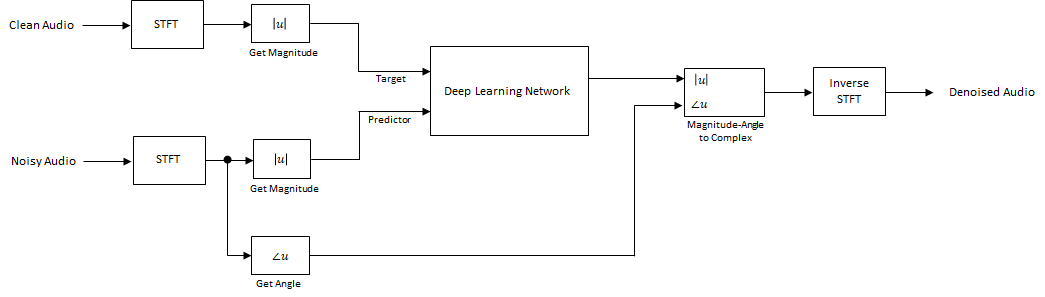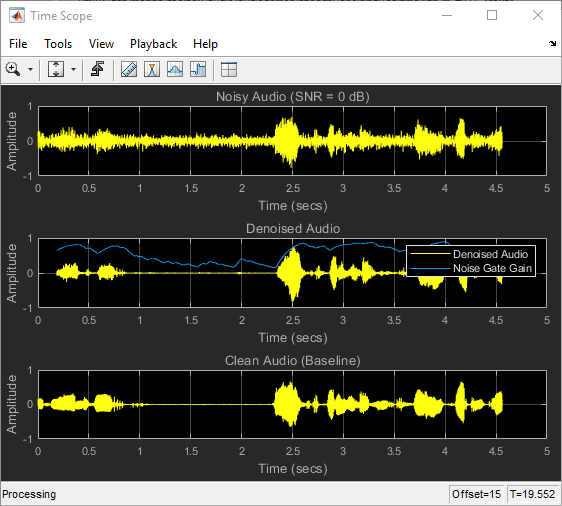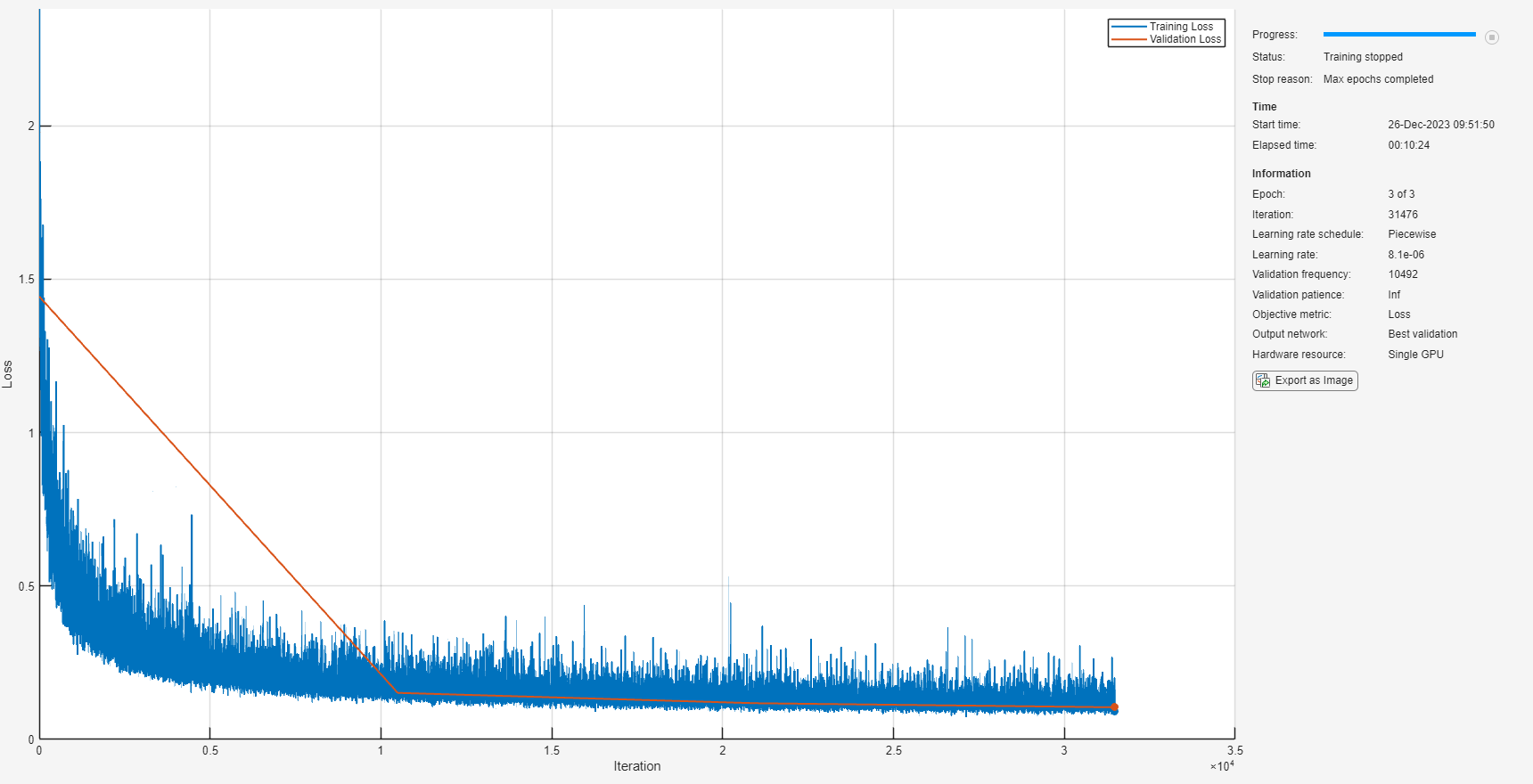# Denoise Speech Using Deep Learning Networks

This example shows how to denoise speech signals using deep learning networks. The example compares two types of networks applied to the same task: fully connected, and convolutional.

### Introduction

The aim of speech denoising is to remove noise from speech signals while enhancing the quality and intelligibility of speech. This example showcases the removal of washing machine noise from speech signals using deep learning networks. The example compares two types of networks applied to the same task: fully connected, and convolutional.

### Problem Summary

Consider the following speech signal sampled at 8 kHz.

```[cleanAudio,fs] = audioread("SpeechDFT-16-8-mono-5secs.wav"); sound(cleanAudio,fs)```

Add washing machine noise to the speech signal. Set the noise power such that the signal-to-noise ratio (SNR) is zero dB.

```noise = audioread("WashingMachine-16-8-mono-1000secs.mp3"); % Extract a noise segment from a random location in the noise file ind = randi(numel(noise) - numel(cleanAudio) + 1,1,1); noiseSegment = noise(ind:ind + numel(cleanAudio) - 1); speechPower = sum(cleanAudio.^2); noisePower = sum(noiseSegment.^2); noisyAudio = cleanAudio + sqrt(speechPower/noisePower)*noiseSegment;```

Listen to the noisy speech signal.

`sound(noisyAudio,fs)`

Visualize the original and noisy signals.

```t = (1/fs)*(0:numel(cleanAudio) - 1); figure(1) tiledlayout(2,1) nexttile plot(t,cleanAudio) title("Clean Audio") grid on nexttile plot(t,noisyAudio) title("Noisy Audio") xlabel("Time (s)") grid on```The objective of speech denoising is to remove the washing machine noise from the speech signal while minimizing undesired artifacts in the output speech.

### Examine the Dataset

This example uses a subset of the Mozilla Common Voice dataset  to train and test the deep learning networks. The data set contains 48 kHz recordings of subjects speaking short sentences. Download the data set and unzip the downloaded file.

```downloadFolder = matlab.internal.examples.downloadSupportFile("audio","commonvoice.zip"); dataFolder = tempdir; unzip(downloadFolder,dataFolder) dataset = fullfile(dataFolder,"commonvoice");```

Use `audioDatastore` to create a datastore for the training set. To speed up the runtime of the example at the cost of performance, set `speedupExample` to `true`.

```adsTrain = audioDatastore(fullfile(dataset,"train"),IncludeSubfolders=true); speedupExample =true; if speedupExample adsTrain = shuffle(adsTrain); adsTrain = subset(adsTrain,1:1000); end```

Use `read` to get the contents of the first file in the datastore.

`[audio,adsTrainInfo] = read(adsTrain);`

Listen to the speech signal.

`sound(audio,adsTrainInfo.SampleRate)`

Plot the speech signal.

```figure(2) t = (1/adsTrainInfo.SampleRate) * (0:numel(audio)-1); plot(t,audio) title("Example Speech Signal") xlabel("Time (s)") grid on```### Deep Learning System Overview

The basic deep learning training scheme is shown below. Note that, since speech generally falls below 4 kHz, you first downsample the clean and noisy audio signals to 8 kHz to reduce the computational load of the network. The predictor and target network signals are the magnitude spectra of the noisy and clean audio signals, respectively. The network's output is the magnitude spectrum of the denoised signal. The regression network uses the predictor input to minimize the mean square error between its output and the input target. The denoised audio is converted back to the time domain using the output magnitude spectrum and the phase of the noisy signal .You transform the audio to the frequency domain using the Short-Time Fourier transform (STFT), with a window length of 256 samples, an overlap of 75%, and a Hamming window. You reduce the size of the spectral vector to 129 by dropping the frequency samples corresponding to negative frequencies (because the time-domain speech signal is real, this does not lead to any information loss). The predictor input consists of 8 consecutive noisy STFT vectors, so that each STFT output estimate is computed based on the current noisy STFT and the 7 previous noisy STFT vectors.### STFT Targets and Predictors

This section illustrates how to generate the target and predictor signals from one training file.

First, define system parameters:

```windowLength = 256; win = hamming(windowLength,"periodic"); overlap = round(0.75*windowLength); fftLength = windowLength; inputFs = 48e3; fs = 8e3; numFeatures = fftLength/2 + 1; numSegments = 8;```

Create a `dsp.SampleRateConverter` object to convert the 48 kHz audio to 8 kHz.

`src = dsp.SampleRateConverter(InputSampleRate=inputFs,OutputSampleRate=fs,Bandwidth=7920);`

Use `read` to get the contents of an audio file from the datastore.

`audio = read(adsTrain);`

Make sure the audio length is a multiple of the sample rate converter decimation factor.

```decimationFactor = inputFs/fs; L = floor(numel(audio)/decimationFactor); audio = audio(1:decimationFactor*L);```

Convert the audio signal to 8 kHz.

```audio = src(audio); reset(src)```

Create a random noise segment from the washing machine noise vector.

```randind = randi(numel(noise) - numel(audio),[1 1]); noiseSegment = noise(randind:randind + numel(audio) - 1);```

Add noise to the speech signal such that the SNR is 0 dB.

```noisePower = sum(noiseSegment.^2); cleanPower = sum(audio.^2); noiseSegment = noiseSegment.*sqrt(cleanPower/noisePower); noisyAudio = audio + noiseSegment;```

Use `stft` to generate magnitude STFT vectors from the original and noisy audio signals.

```cleanSTFT = stft(audio,Window=win,OverlapLength=overlap,fftLength=fftLength); cleanSTFT = abs(cleanSTFT(numFeatures-1:end,:)); noisySTFT = stft(noisyAudio,Window=win,OverlapLength=overlap,fftLength=fftLength); noisySTFT = abs(noisySTFT(numFeatures-1:end,:));```

Generate the 8-segment training predictor signals from the noisy STFT. The overlap between consecutive predictors is 7 segments.

```noisySTFT = [noisySTFT(:,1:numSegments - 1),noisySTFT]; stftSegments = zeros(numFeatures,numSegments,size(noisySTFT,2) - numSegments + 1); for index = 1:size(noisySTFT,2) - numSegments + 1 stftSegments(:,:,index) = noisySTFT(:,index:index + numSegments - 1); end```

Set the targets and predictors. The last dimension of both variables corresponds to the number of distinct predictor/target pairs generated by the audio file. Each predictor is 129-by-8, and each target is 129-by-1.

```targets = cleanSTFT; size(targets)```
```ans = 1×2 129 544 ```
```predictors = stftSegments; size(predictors)```
```ans = 1×3 129 8 544 ```

### Extract Features Using Tall Arrays

To speed up processing, extract feature sequences from the speech segments of all audio files in the datastore using tall arrays. Unlike in-memory arrays, tall arrays typically remain unevaluated until you call the `gather` function. This deferred evaluation enables you to work quickly with large data sets. When you eventually request output using `gather`, MATLAB combines the queued calculations where possible and takes the minimum number of passes through the data. If you have Parallel Computing Toolbox™, you can use tall arrays in your local MATLAB session, or on a local parallel pool. You can also run tall array calculations on a cluster if you have MATLAB® Parallel Server™ installed.

First, convert the datastore to a tall array.

```reset(adsTrain) T = tall(adsTrain)```
```Starting parallel pool (parpool) using the 'local' profile ... Connected to the parallel pool (number of workers: 6). T = M×1 tall cell array {234480×1 double} {210288×1 double} {282864×1 double} {292080×1 double} {410736×1 double} {303600×1 double} {326640×1 double} {233328×1 double} : : : : ```

The display indicates that the number of rows (corresponding to the number of files in the datastore), M, is not yet known. M is a placeholder until the calculation completes.

Extract the target and predictor magnitude STFT from the tall table. This action creates new tall array variables to use in subsequent calculations. The function `HelperGenerateSpeechDenoisingFeatures` performs the steps already highlighted in the STFT Targets and Predictors section. The `cellfun` command applies `HelperGenerateSpeechDenoisingFeatures` to the contents of each audio file in the datastore.

`[targets,predictors] = cellfun(@(x)HelperGenerateSpeechDenoisingFeatures(x,noise,src),T,UniformOutput=false);`

Use `gather` to evaluate the targets and predictors.

`[targets,predictors] = gather(targets,predictors);`
```Evaluating tall expression using the Parallel Pool 'local': - Pass 1 of 1: Completed in 52 sec Evaluation completed in 1 min 53 sec ```

It is good practice to normalize all features to zero mean and unity standard deviation.

Compute the mean and standard deviation of the predictors and targets, respectively, and use them to normalize the data.

```predictors = cat(3,predictors{:}); noisyMean = mean(predictors(:)); noisyStd = std(predictors(:)); predictors(:) = (predictors(:) - noisyMean)/noisyStd; targets = cat(2,targets{:}); cleanMean = mean(targets(:)); cleanStd = std(targets(:)); targets(:) = (targets(:) - cleanMean)/cleanStd;```

Reshape predictors and targets to the dimensions expected by the deep learning networks.

```predictors = reshape(predictors,size(predictors,1),size(predictors,2),1,size(predictors,3)); targets = reshape(targets,1,1,size(targets,1),size(targets,2));```

You will use 1% of the data for validation during training. Validation is useful to detect scenarios where the network is overfitting the training data.

Randomly split the data into training and validation sets.

```inds = randperm(size(predictors,4)); L = round(0.99*size(predictors,4)); trainPredictors = predictors(:,:,:,inds(1:L)); trainTargets = targets(:,:,:,inds(1:L)); validatePredictors = predictors(:,:,:,inds(L+1:end)); validateTargets = targets(:,:,:,inds(L+1:end));```

### Speech Denoising with Fully Connected Layers

You first consider a denoising network comprised of fully connected layers. Each neuron in a fully connected layer is connected to all activations from the previous layer. A fully connected layer multiplies the input by a weight matrix and then adds a bias vector. The dimensions of the weight matrix and bias vector are determined by the number of neurons in the layer and the number of activations from the previous layer.Define the layers of the network. Specify the input size to be images of size `NumFeatures`-by-`NumSegments` (129-by-8 in this example). Define two hidden fully connected layers, each with 1024 neurons. Since purely linear systems, follow each hidden fully connected layer with a Rectified Linear Unit (ReLU) layer. The batch normalization layers normalize the means and standard deviations of the outputs. Add a fully connected layer with 129 neurons, followed by a regression layer.

```layers = [ imageInputLayer([numFeatures,numSegments]) fullyConnectedLayer(1024) batchNormalizationLayer reluLayer fullyConnectedLayer(1024) batchNormalizationLayer reluLayer fullyConnectedLayer(numFeatures) regressionLayer ];```

Next, specify the training options for the network. Set `MaxEpochs` to `3` so that the network makes 3 passes through the training data. Set `MiniBatchSize` of `128` so that the network looks at 128 training signals at a time. Specify `Plots` as `"training-progress"` to generate plots that show the training progress as the number of iterations increases. Set `Verbose` to `false` to disable printing the table output that corresponds to the data shown in the plot into the command line window. Specify `Shuffle` as `"every-epoch"` to shuffle the training sequences at the beginning of each epoch. Specify `LearnRateSchedule` to `"piecewise"` to decrease the learning rate by a specified factor (0.9) every time a certain number of epochs (1) has passed. Set `ValidationData` to the validation predictors and targets. Set `ValidationFrequency` such that the validation mean square error is computed once per epoch. This example uses the adaptive moment estimation (Adam) solver.

```miniBatchSize = 128; options = trainingOptions("adam", ... MaxEpochs=3, ... InitialLearnRate=1e-5,... MiniBatchSize=miniBatchSize, ... Shuffle="every-epoch", ... Plots="training-progress", ... Verbose=false, ... ValidationFrequency=floor(size(trainPredictors,4)/miniBatchSize), ... LearnRateSchedule="piecewise", ... LearnRateDropFactor=0.9, ... LearnRateDropPeriod=1, ... ValidationData={validatePredictors,validateTargets});```

Train the network with the specified training options and layer architecture using `trainNetwork`. Because the training set is large, the training process can take several minutes. To download and load a pre-trained network instead of training a network from scratch, set `downloadPretrainedSystem` to `true`.

```downloadPretrainedSystem =false; if downloadPretrainedSystem downloadFolder = matlab.internal.examples.downloadSupportFile("audio","SpeechDenoising.zip"); dataFolder = tempdir; unzip(downloadFolder,dataFolder) netFolder = fullfile(dataFolder,"SpeechDenoising"); s = load(fullfile(netFolder,"denoisenet.mat")); denoiseNetFullyConnected = s.denoiseNetFullyConnected; cleanMean = s.cleanMean; cleanStd = s.cleanStd; noisyMean = s.noisyMean; noisyStd = s.noisyStd; else denoiseNetFullyConnected = trainNetwork(trainPredictors,trainTargets,layers,options); end```

Count the number of weights in the fully connected layers of the network.

```numWeights = 0; for index = 1:numel(denoiseNetFullyConnected.Layers) if isa(denoiseNetFullyConnected.Layers(index),"nnet.cnn.layer.FullyConnectedLayer") numWeights = numWeights + numel(denoiseNetFullyConnected.Layers(index).Weights); end end disp("Number of weights = " + numWeights);```
```Number of weights = 2237440 ```

### Speech Denoising with Convolutional Layers

Consider a network that uses convolutional layers instead of fully connected layers . A 2-D convolutional layer applies sliding filters to the input. The layer convolves the input by moving the filters along the input vertically and horizontally and computing the dot product of the weights and the input, and then adding a bias term. Convolutional layers typically consist of fewer parameters than fully connected layers.

Define the layers of the fully convolutional network described in , comprising 16 convolutional layers. The first 15 convolutional layers are groups of 3 layers, repeated 5 times, with filter widths of 9, 5, and 9, and number of filters of 18, 30 and 8, respectively. The last convolutional layer has a filter width of 129 and 1 filter. In this network, convolutions are performed in only one direction (along the frequency dimension), and the filter width along the time dimension is set to 1 for all layers except the first one. Similar to the fully connected network, convolutional layers are followed by ReLu and batch normalization layers.

```layers = [imageInputLayer([numFeatures,numSegments]) convolution2dLayer([9 8],18,Stride=[1 100],Padding="same") batchNormalizationLayer reluLayer repmat( ... [convolution2dLayer([5 1],30,Stride=[1 100],Padding="same") batchNormalizationLayer reluLayer convolution2dLayer([9 1],8,Stride=[1 100],Padding="same") batchNormalizationLayer reluLayer convolution2dLayer([9 1],18,Stride=[1 100],Padding="same") batchNormalizationLayer reluLayer],4,1) convolution2dLayer([5 1],30,Stride=[1 100],Padding="same") batchNormalizationLayer reluLayer convolution2dLayer([9 1],8,Stride=[1 100],Padding="same") batchNormalizationLayer reluLayer convolution2dLayer([129 1],1,Stride=[1 100],Padding="same") regressionLayer ];```

The training options are identical to the options for the fully connected network, except that the dimensions of the validation target signals are permuted to be consistent with the dimensions expected by the regression layer.

```options = trainingOptions("adam", ... MaxEpochs=3, ... InitialLearnRate=1e-5, ... MiniBatchSize=miniBatchSize, ... Shuffle="every-epoch", ... Plots="training-progress", ... Verbose=false, ... ValidationFrequency=floor(size(trainPredictors,4)/miniBatchSize), ... LearnRateSchedule="piecewise", ... LearnRateDropFactor=0.9, ... LearnRateDropPeriod=1, ... ValidationData={validatePredictors,permute(validateTargets,[3 1 2 4])});```

Train the network with the specified training options and layer architecture using `trainNetwork`. Because the training set is large, the training process can take several minutes. To download and load a pre-trained network instead of training a network from scratch, set `downloadPretrainedSystem` to `true`.

```downloadPretrainedSystem =false; if downloadPretrainedSystem downloadFolder = matlab.internal.examples.downloadSupportFile("audio","SpeechDenoising.zip"); dataFolder = tempdir; unzip(downloadFolder,dataFolder) netFolder = fullfile(dataFolder,"SpeechDenoising"); s = load(fullfile(netFolder,"denoisenet.mat")); denoiseNetFullyConvolutional = s.denoiseNetFullyConvolutional; cleanMean = s.cleanMean; cleanStd = s.cleanStd; noisyMean = s.noisyMean; noisyStd = s.noisyStd; else denoiseNetFullyConvolutional = trainNetwork(trainPredictors,permute(trainTargets,[3 1 2 4]),layers,options); end```

Count the number of weights in the fully connected layers of the network.

```numWeights = 0; for index = 1:numel(denoiseNetFullyConvolutional.Layers) if isa(denoiseNetFullyConvolutional.Layers(index),"nnet.cnn.layer.Convolution2DLayer") numWeights = numWeights + numel(denoiseNetFullyConvolutional.Layers(index).Weights); end end disp("Number of weights in convolutional layers = " + numWeights);```
```Number of weights in convolutional layers = 31812 ```

### Test the Denoising Networks

Read in the test data set.

`adsTest = audioDatastore(fullfile(dataset,"test"),IncludeSubfolders=true);`

Read the contents of a file from the datastore.

`[cleanAudio,adsTestInfo] = read(adsTest);`

Make sure the audio length is a multiple of the sample rate converter decimation factor.

```L = floor(numel(cleanAudio)/decimationFactor); cleanAudio = cleanAudio(1:decimationFactor*L);```

Convert the audio signal to 8 kHz.

```cleanAudio = src(cleanAudio); reset(src)```

In this testing stage, you corrupt speech with washing machine noise not used in the training stage.

`noise = audioread("WashingMachine-16-8-mono-200secs.mp3");`

Create a random noise segment from the washing machine noise vector.

```randind = randi(numel(noise) - numel(cleanAudio), [1 1]); noiseSegment = noise(randind:randind + numel(cleanAudio) - 1);```

Add noise to the speech signal such that the SNR is 0 dB.

```noisePower = sum(noiseSegment.^2); cleanPower = sum(cleanAudio.^2); noiseSegment = noiseSegment.*sqrt(cleanPower/noisePower); noisyAudio = cleanAudio + noiseSegment;```

Use `stft` to generate magnitude STFT vectors from the noisy audio signals.

```noisySTFT = stft(noisyAudio,Window=win,OverlapLength=overlap,fftLength=fftLength); noisyPhase = angle(noisySTFT(numFeatures-1:end,:)); noisySTFT = abs(noisySTFT(numFeatures-1:end,:));```

Generate the 8-segment training predictor signals from the noisy STFT. The overlap between consecutive predictors is 7 segments.

```noisySTFT = [noisySTFT(:,1:numSegments-1) noisySTFT]; predictors = zeros(numFeatures,numSegments,size(noisySTFT,2) - numSegments + 1); for index = 1:(size(noisySTFT,2) - numSegments + 1) predictors(:,:,index) = noisySTFT(:,index:index + numSegments - 1); end```

Normalize the predictors by the mean and standard deviation computed in the training stage.

`predictors(:) = (predictors(:) - noisyMean)/noisyStd;`

Compute the denoised magnitude STFT by using `predict` with the two trained networks.

```predictors = reshape(predictors,[numFeatures,numSegments,1,size(predictors,3)]); STFTFullyConnected = predict(denoiseNetFullyConnected,predictors); STFTFullyConvolutional = predict(denoiseNetFullyConvolutional,predictors);```

Scale the outputs by the mean and standard deviation used in the training stage.

```STFTFullyConnected(:) = cleanStd*STFTFullyConnected(:) + cleanMean; STFTFullyConvolutional(:) = cleanStd*STFTFullyConvolutional(:) + cleanMean;```

Convert the one-sided STFT to a centered STFT.

```STFTFullyConnected = (STFTFullyConnected.').*exp(1j*noisyPhase); STFTFullyConnected = [conj(STFTFullyConnected(end-1:-1:2,:));STFTFullyConnected]; STFTFullyConvolutional = squeeze(STFTFullyConvolutional).*exp(1j*noisyPhase); STFTFullyConvolutional = [conj(STFTFullyConvolutional(end-1:-1:2,:));STFTFullyConvolutional];```

Compute the denoised speech signals. `istft` performs the inverse STFT. Use the phase of the noisy STFT vectors to reconstruct the time-domain signal.

```denoisedAudioFullyConnected = istft(STFTFullyConnected,Window=win,OverlapLength=overlap,fftLength=fftLength,ConjugateSymmetric=true); denoisedAudioFullyConvolutional = istft(STFTFullyConvolutional,Window=win,OverlapLength=overlap,fftLength=fftLength,ConjugateSymmetric=true);```

Plot the clean, noisy and denoised audio signals.

```t = (1/fs)*(0:numel(denoisedAudioFullyConnected)-1); figure(3) tiledlayout(4,1) nexttile plot(t,cleanAudio(1:numel(denoisedAudioFullyConnected))) title("Clean Speech") grid on nexttile plot(t,noisyAudio(1:numel(denoisedAudioFullyConnected))) title("Noisy Speech") grid on nexttile plot(t,denoisedAudioFullyConnected) title("Denoised Speech (Fully Connected Layers)") grid on nexttile plot(t,denoisedAudioFullyConvolutional) title("Denoised Speech (Convolutional Layers)") grid on xlabel("Time (s)")```Plot the clean, noisy, and denoised spectrograms.

```h = figure(4); tiledlayout(4,1) nexttile spectrogram(cleanAudio,win,overlap,fftLength,fs); title("Clean Speech") grid on nexttile spectrogram(noisyAudio,win,overlap,fftLength,fs); title("Noisy Speech") grid on nexttile spectrogram(denoisedAudioFullyConnected,win,overlap,fftLength,fs); title("Denoised Speech (Fully Connected Layers)") grid on nexttile spectrogram(denoisedAudioFullyConvolutional,win,overlap,fftLength,fs); title("Denoised Speech (Convolutional Layers)") grid on p = get(h,"Position"); set(h,"Position",[p(1) 65 p(3) 800]);```Listen to the noisy speech.

`sound(noisyAudio,fs)`

Listen to the denoised speech from the network with fully connected layers.

`sound(denoisedAudioFullyConnected,fs)`

Listen to the denoised speech from the network with convolutional layers.

`sound(denoisedAudioFullyConvolutional,fs)`

Listen to clean speech.

`sound(cleanAudio,fs)`

You can test more files from the datastore by calling `testDenoisingNets`. The function produces the time-domain and frequency-domain plots highlighted above, and also returns the clean, noisy, and denoised audio signals.

`[cleanAudio,noisyAudio,denoisedAudioFullyConnected,denoisedAudioFullyConvolutional] = testDenoisingNets(adsTest,denoiseNetFullyConnected,denoiseNetFullyConvolutional,noisyMean,noisyStd,cleanMean,cleanStd);`### Real-Time Application

The procedure in the previous section passes the entire spectrum of the noisy signal to `predict`. This is not suitable for real-time applications where low latency is a requirement.

Run `speechDenoisingRealtimeApp` for an example of how to simulate a streaming, real-time version of the denoising network. The app uses the network with fully connected layers. The audio frame length is equal to the STFT hop size, which is 0.25 * 256 = 64 samples.

`speechDenoisingRealtimeApp` launches a User Interface (UI) designed to interact with the simulation. The UI enables you to tune parameters and the results are reflected in the simulation instantly. You can also enable/disable a noise gate that operates on the denoised output to further reduce the noise, as well as tune the attack time, release time, and threshold of the noise gate. You can listen to the noisy, clean or denoised audio from the UI.The scope plots the clean, noisy and denoised signals, as well as the gain of the noise gate.### References

 "Experiments on Deep Learning for Speech Denoising", Ding Liu, Paris Smaragdis, Minje Kim, INTERSPEECH, 2014.

 "A Fully Convolutional Neural Network for Speech Enhancement", Se Rim Park, Jin Won Lee, INTERSPEECH, 2017.